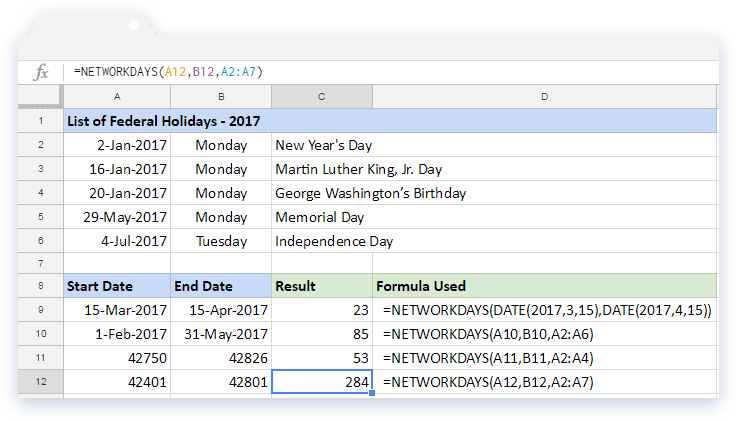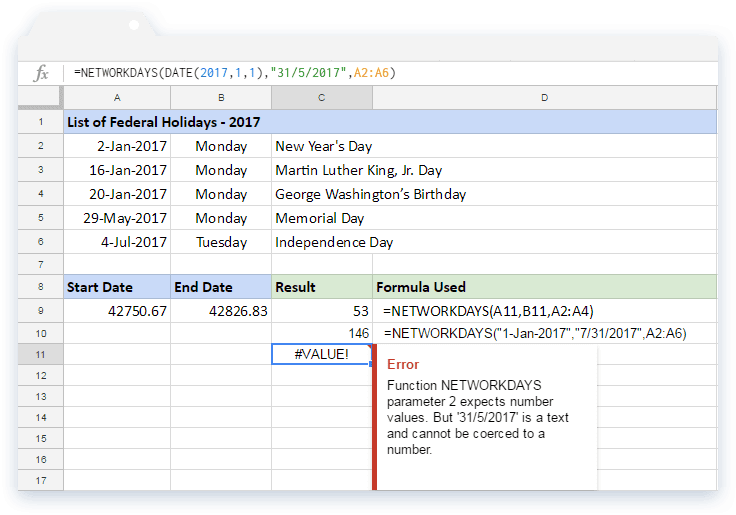# How to use the NETWORKDAYS formula in Google SheetsIn Google Sheets application, if we need to know the number of net working days between two specified dates, the NETWORKDAYS formula can be our companion.

### Syntax

NETWORKDAYS(start_date, end_date, [holidays])

• start_date – the starting date in the period from which the formula calculates the number of net working days. This date, if not a holiday, is considered for counting as a working day.
• end_date – the ending date in the period until which the formula calculates the number of net working days. Like start_date, this date too, if not a holiday, is considered for counting as a working day.
• holidays – is an optional parameter that expects either an array or an address reference to the range of cells that consists of holiday dates.

### Usage: NETWORKDAYS Formula

Let us try our hands on this formula. Please consider the snapshot below. We have a sample list of holiday dates from A2 to A6.In the first example case, the start_date and end_date parameters take outputs from two different DATE() functions, evaluated to 15-Mar-2017 and 15-Apr-2017 respectively. And, we ignored the holiday’s parameter altogether. Therefore, the NETWORKDAYS formula calculates the resulting number of work days falling between the start_date and end_date. It does so by ignoring the Saturdays and Sundays but does include the start and end dates

The second example is pretty similar. Except, the start_date and the end_dateparameters are references to the cells that has dates. Also, we have given a range reference to the list of holidays for the third parameter. So it counts the net working days after ignoring Saturdays, Sundays, and the list of holidays that fall between the start_date and end_date.

Unlike the previous two cases, the input values for start_date and end_dateparameters are absolute numbers for the next two examples. Nevertheless, the formula’s working remains the same.

##### Cases of interest

Let us experiment a little further and input text based date values for the start_dateand start_date parameter.In the first example, the formula truncates the decimal values and uses only the integer values and accordingly evaluates the count. Of course, it cannot accept negative numbers, as there is no date interpretation for such values.

From the next two examples, we will see that the NETWORKDAYS formula accepts input date strings in the “dd-mmm-yyyy” and “mm-dd-yyyy” formats. But it will throw up an error with “dd-mm-yyyy” date format strings.

### NETWORKDAYS formula

And there you go! Use the NETWORKDAYS formula in Google Sheets to calculate the number of net working days between two specified dates.

If you’d like to learn more about the various formulas of Google Sheets, why not take a look at our blog post on the DAYS360 formula in Google Sheets.

Alternatively, check out related blog posts below!